SBAS512F April   2011  – February 2020

PRODUCTION DATA.

1. Features
2. Applications
3. Description
1.     Device Images
4. Revision History
5. Pin Configuration and Functions
6. Specifications
7. Detailed Description
1. 7.1 Overview
2. 7.2 Functional Block Diagram
3. 7.3 Feature Description
4. 7.4 Device Functional Modes
8. Application and Implementation
1. 8.1 Application Information
2. 8.2 Typical Application
1. 8.2.1 Frequency Inverter Application
2. 8.2.2 Example of a Resolver-Based Motor Control Analog Front End
3. 8.2.3 Isolated Voltage Sensing
9. Power Supply Recommendations
10. 10Layout
11. 11Device and Documentation Support
12. 12Mechanical, Packaging, and Orderable Information

• DWV|8
• DW|16
• DW|16

### 6.12 Typical Characteristics

at AVDD = 5 V, DVDD = 3.3 V, VINP = –250 mV to 250 mV, VINN = 0 V, and sinc3 filter with OSR = 256 (unless otherwise noted)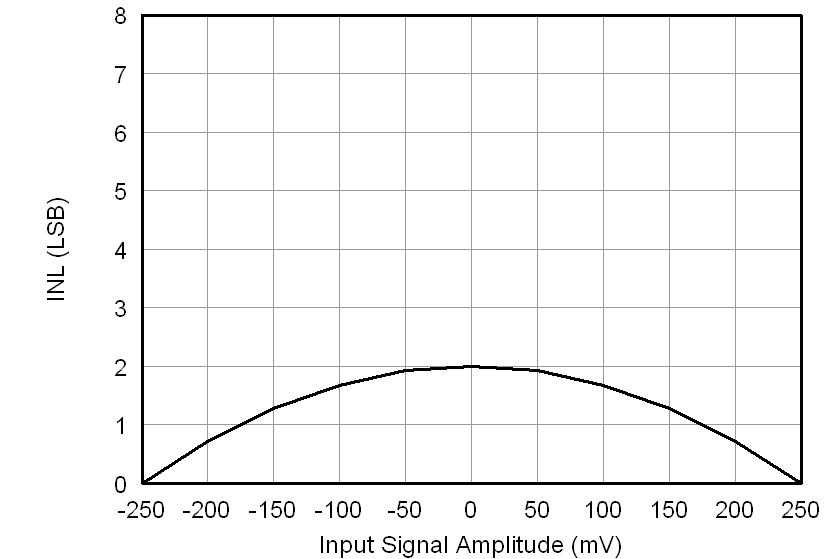Figure 4. Integral Nonlinearity vs Input Signal Amplitude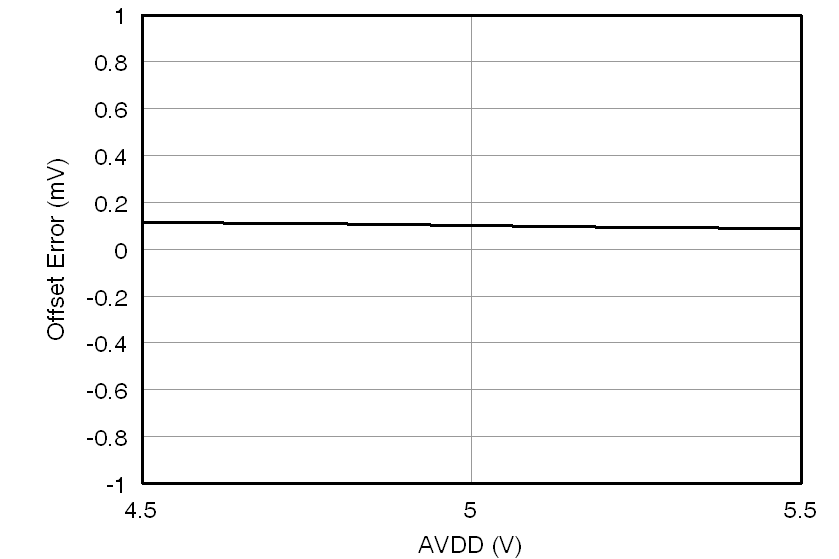Figure 6. Offset Error vs Analog Supply Voltage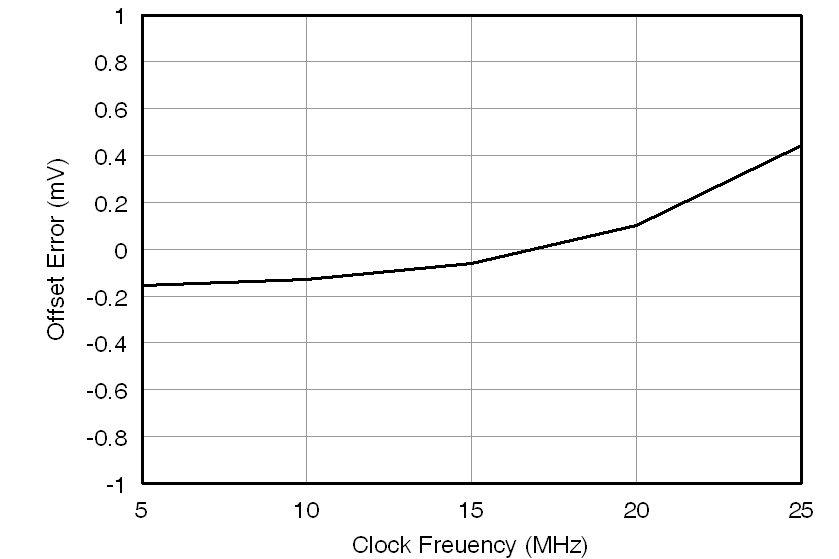Figure 8. Offset Error vs Clock Frequency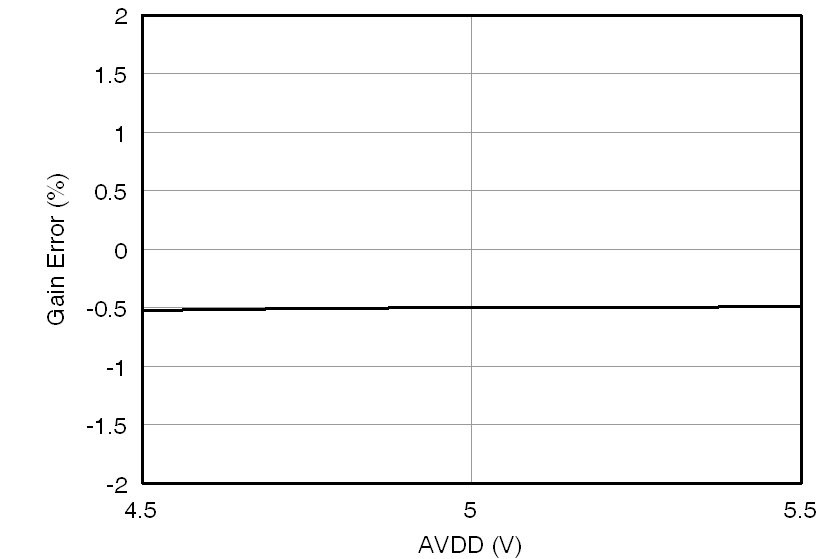Figure 10. Gain Error vs Analog Supply Voltage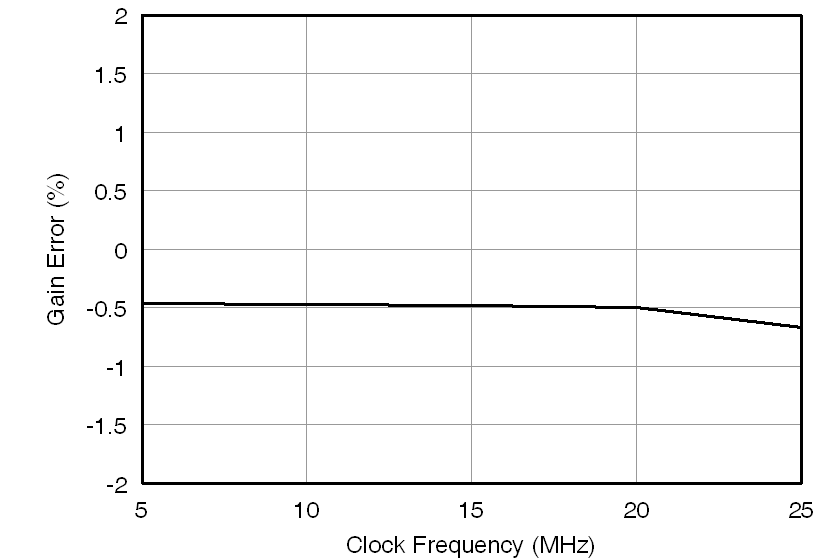Figure 12. Gain Error vs Clock Frequency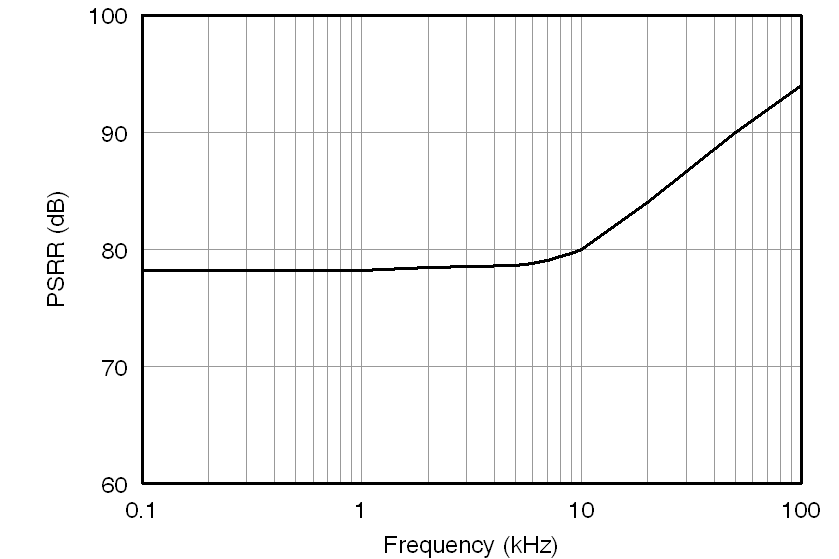Figure 14. Power-Supply Rejection Ratio vs Frequency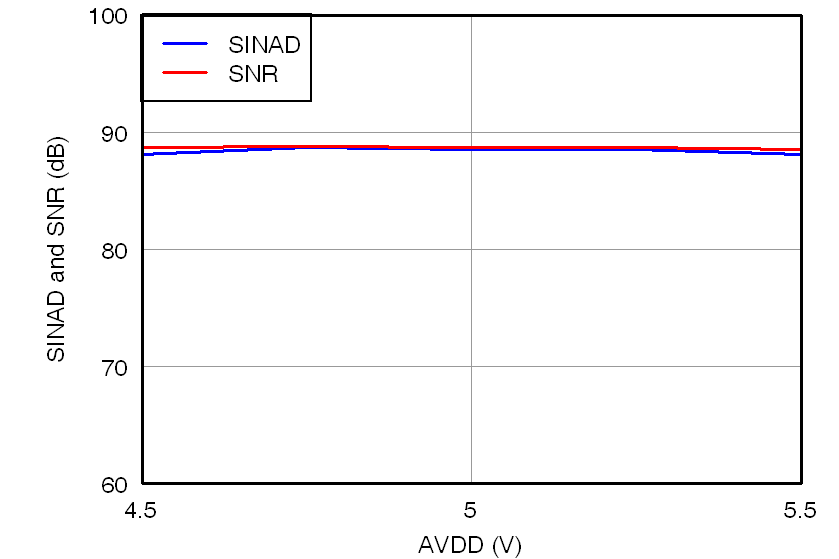Figure 16. SINAD and SNR vs Analog Supply Voltage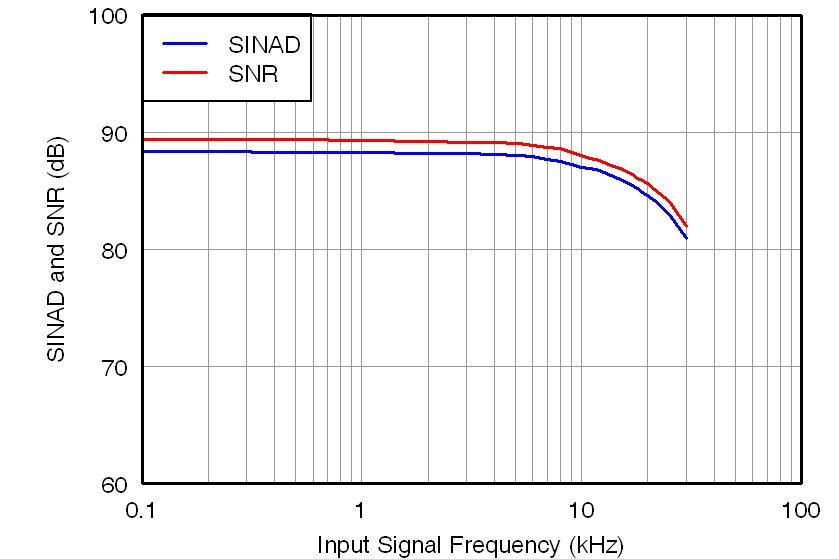Figure 18. SINAD and SNR vs Input Signal Frequency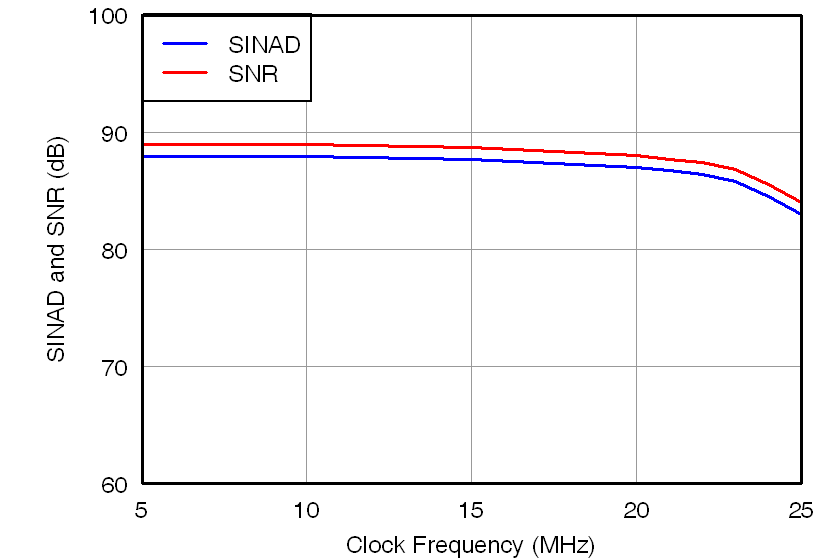Figure 20. SINAD and SNR vs Clock Frequency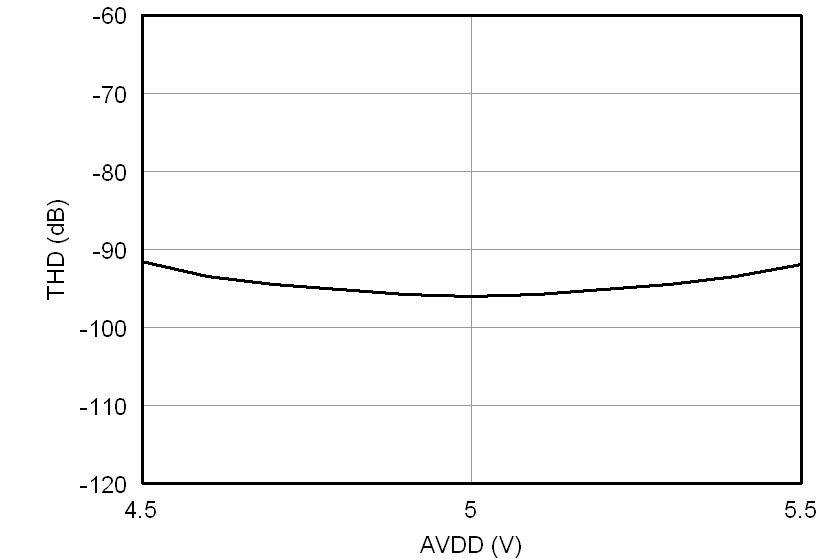Figure 22. Total Harmonic Distortion vs Analog Supply VoltageFigure 24. Total Harmonic Distortion vs Input Signal Frequency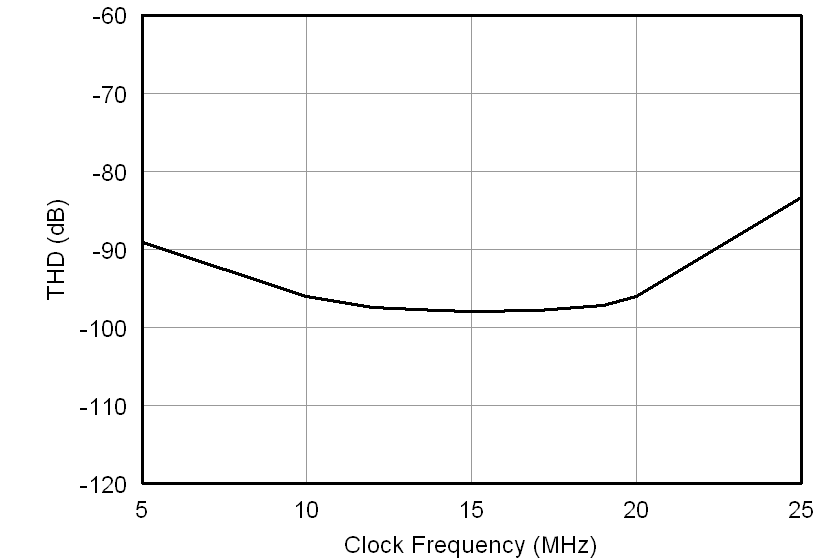Figure 26. Total Harmonic Distortion vs Clock Frequency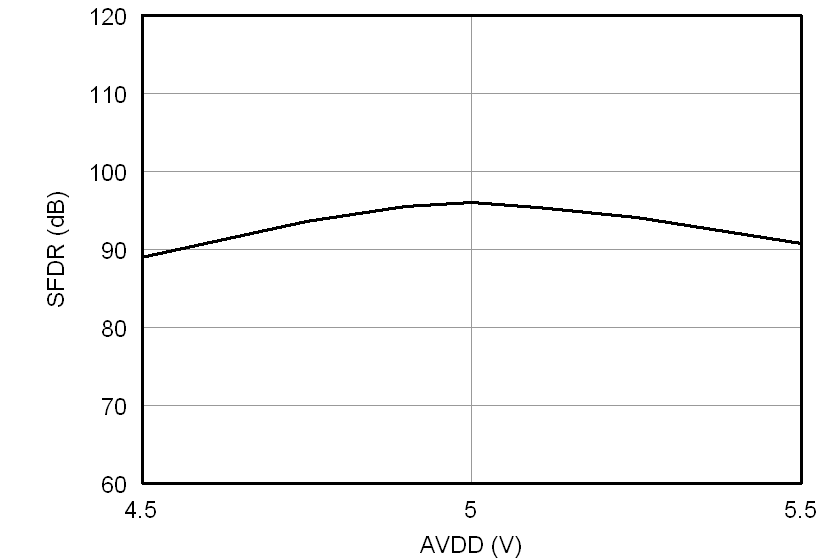Figure 28. Spurious-Free Dynamic Range vs Analog Supply VoltageFigure 30. Spurious-Free Dynamic Range vs Input Signal Frequency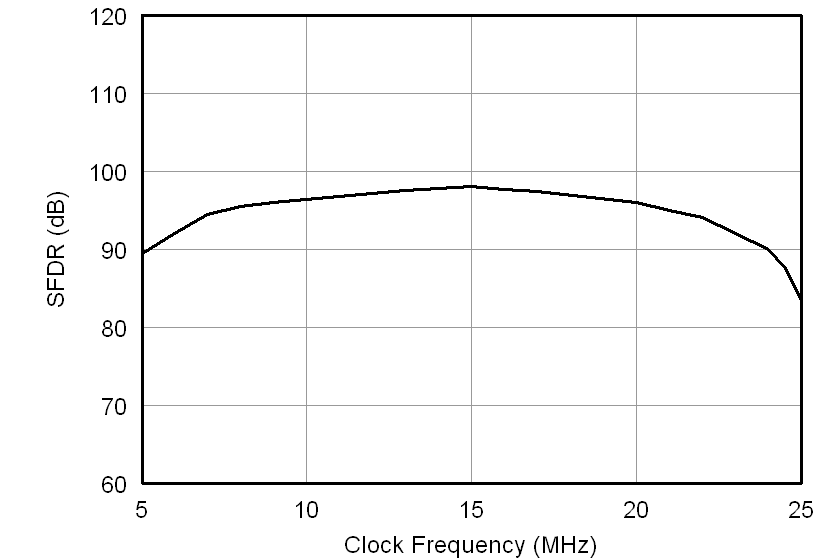Figure 32. Spurious-Free Dynamic Range vs Clock Frequency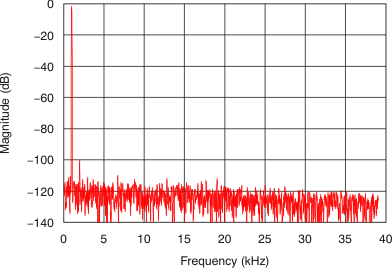Figure 34. Frequency Spectrum (4096 Point FFT, fIN = 1 kHz, 0.56 VPP)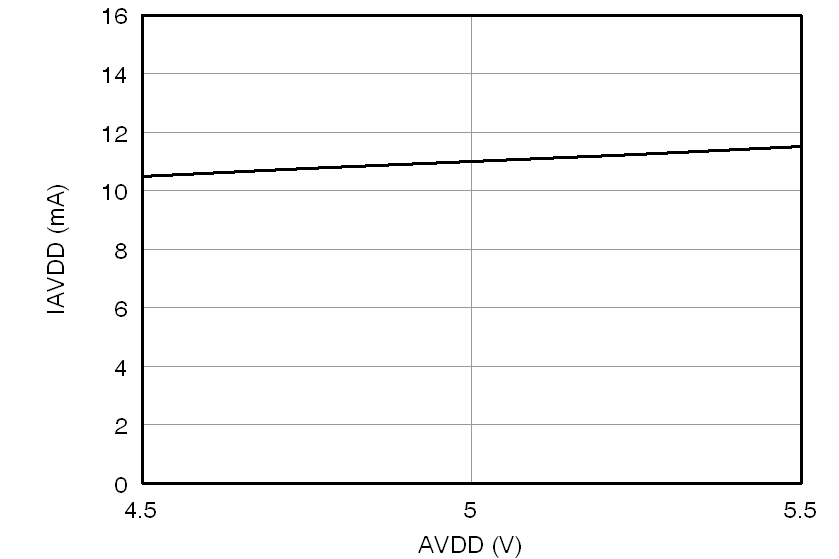Figure 36. Analog Supply Current vs Analog Supply Voltage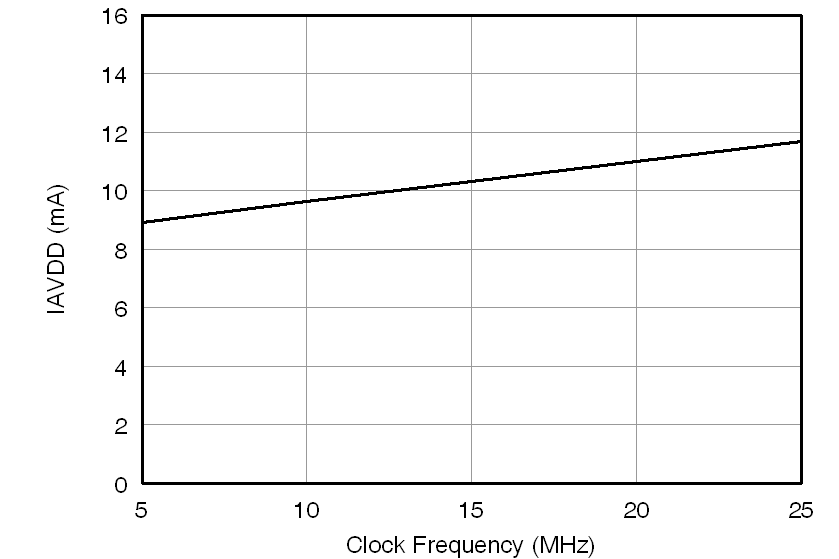Figure 38. Analog Supply Current vs Clock Frequency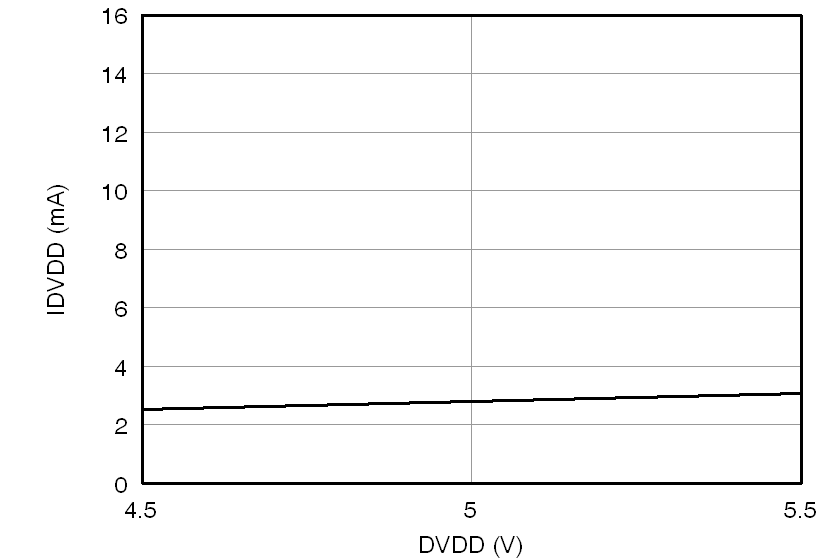Figure 40. Digital Supply Current vs Digital Supply Voltage (5 V)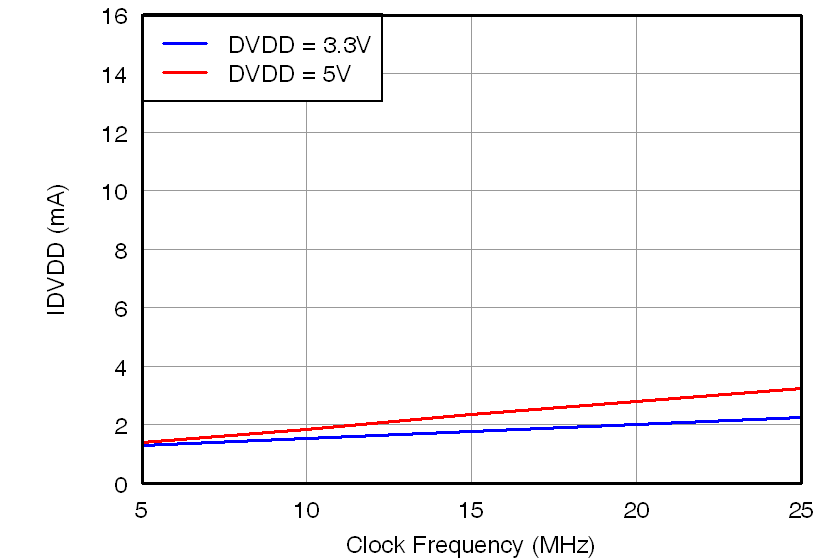Figure 42. Digital Supply Current vs Clock Frequency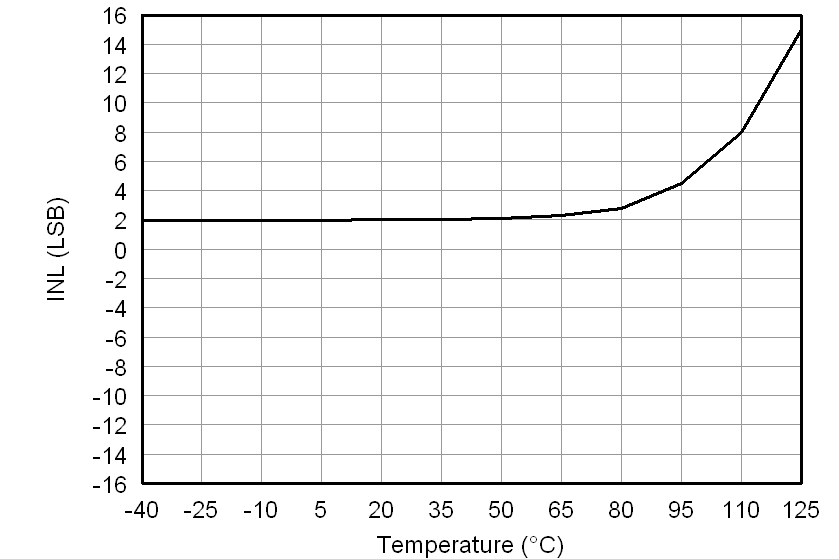Figure 5. Integral Nonlinearity vs Temperature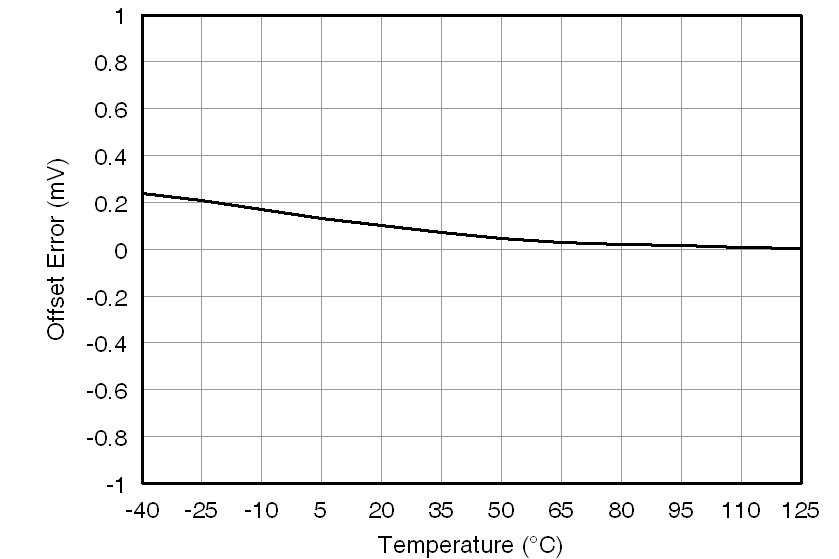Figure 7. Offset Error vs Temperature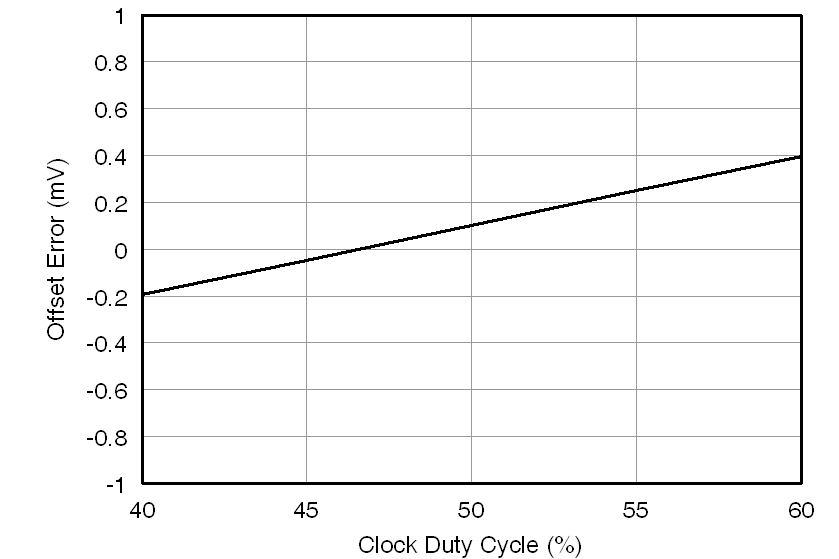Figure 9. Offset Error vs Clock Duty Cycle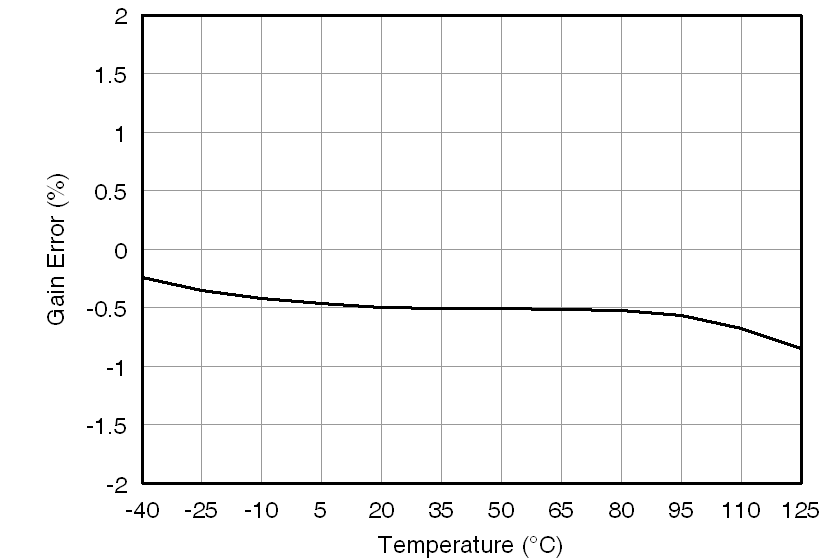Figure 11. Gain Error vs TemperatureFigure 13. Gain Error vs Clock Duty Cycle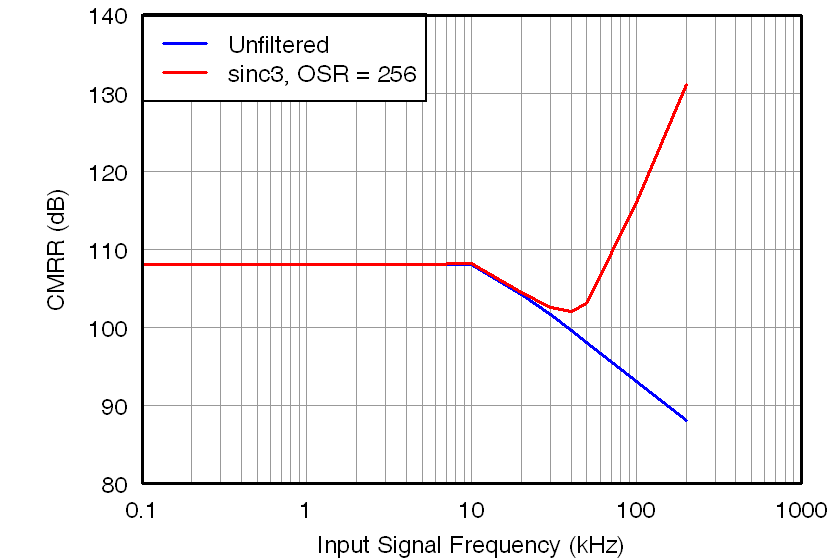Figure 15. Common-Mode Rejection Ratio vs Input Signal Frequency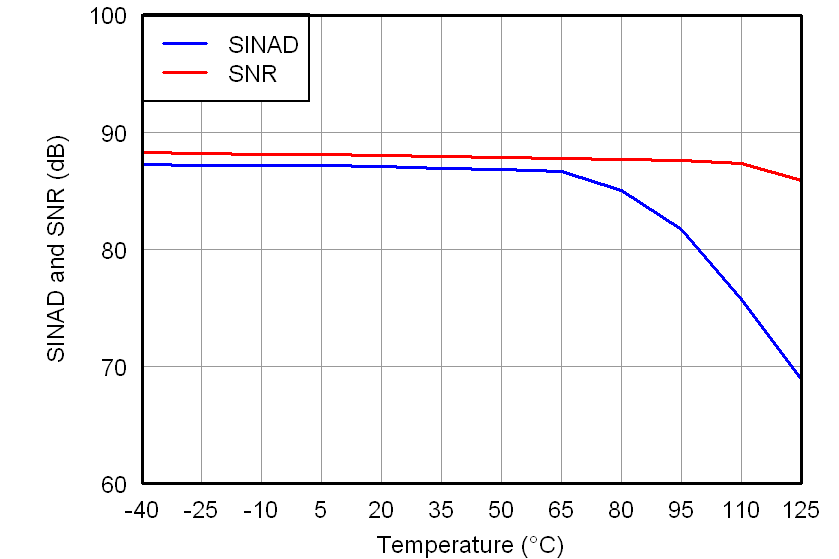Figure 17. SINAD and SNR vs Temperature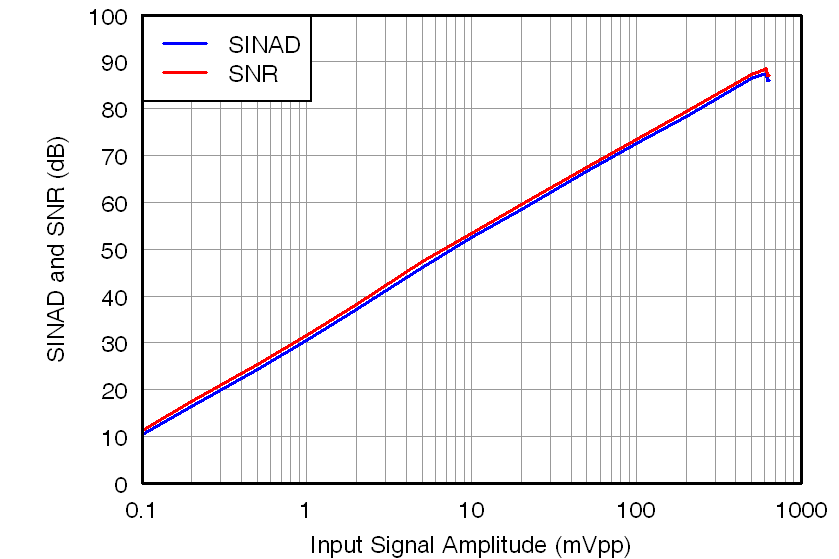Figure 19. SINAD and SNR vs Input Signal AmplitudeFigure 21. SINAD and SNR vs Clock Duty Cycle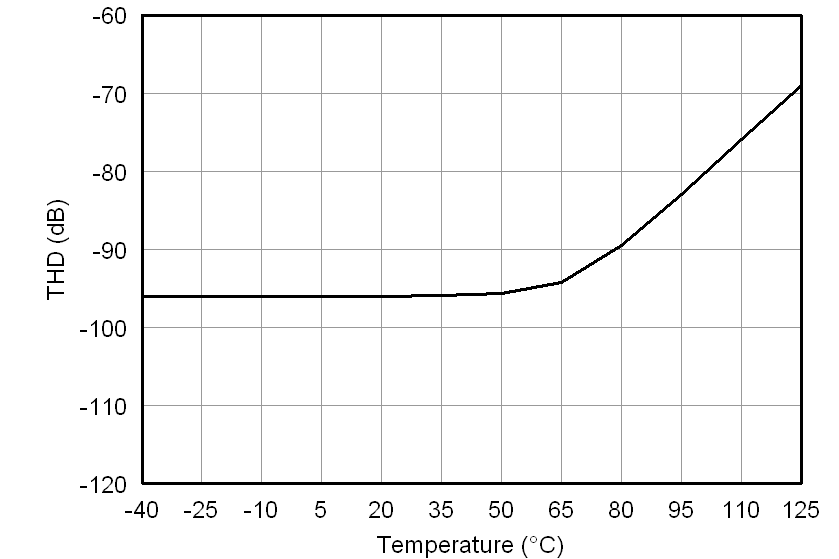Figure 23. Total Harmonic Distortion vs Temperature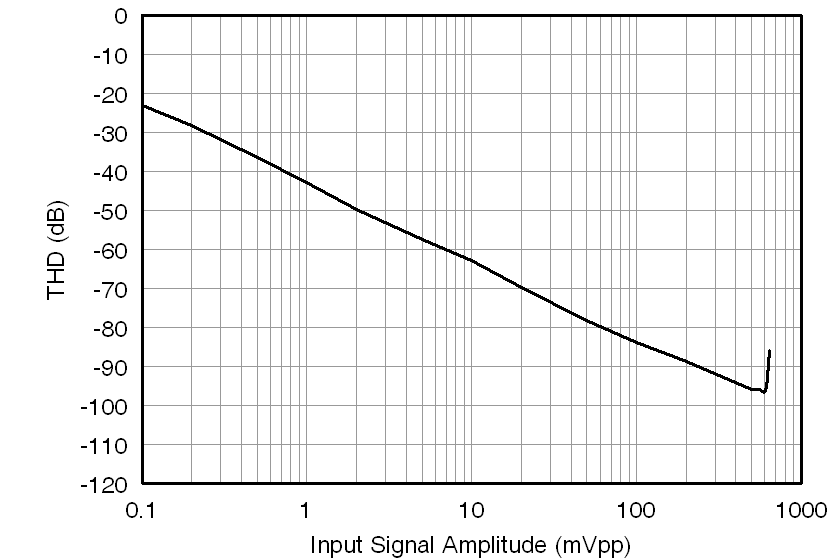Figure 25. Total Harmonic Distortion vs Input Signal Amplitude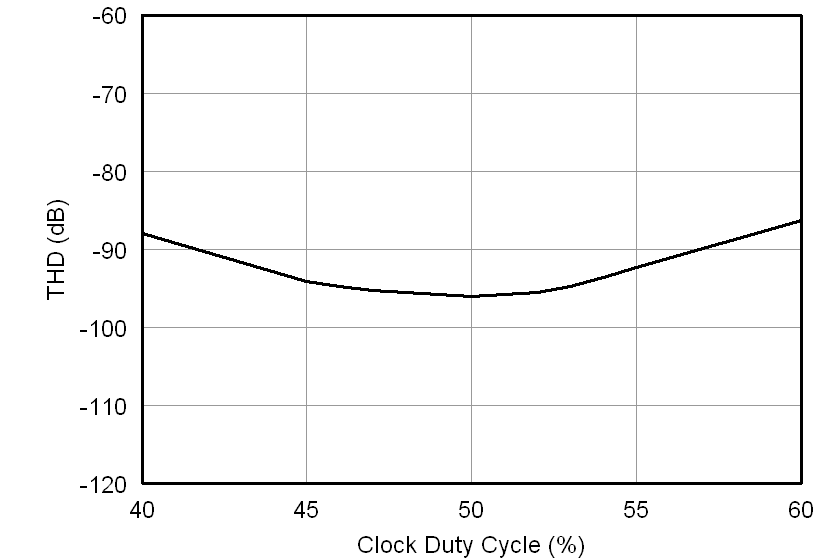Figure 27. Total Harmonic Distortion vs Clock Duty Cycle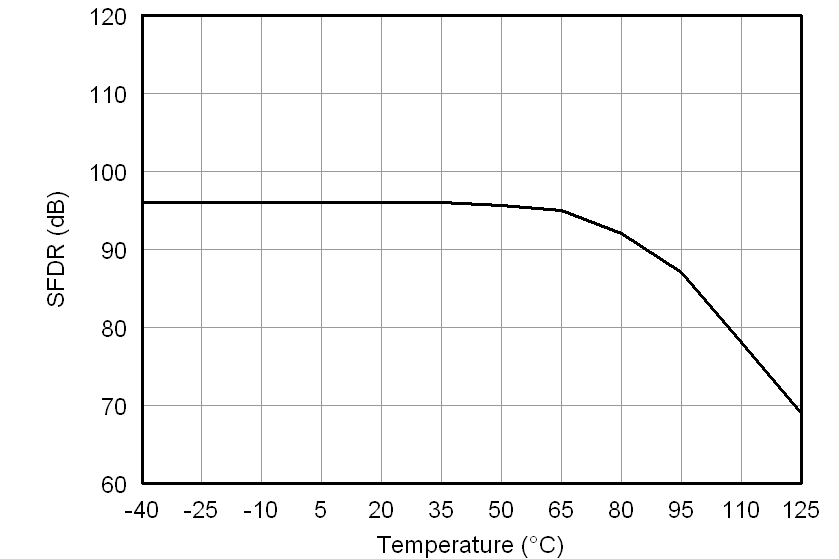Figure 29. Spurious-Free Dynamic Range vs Temperature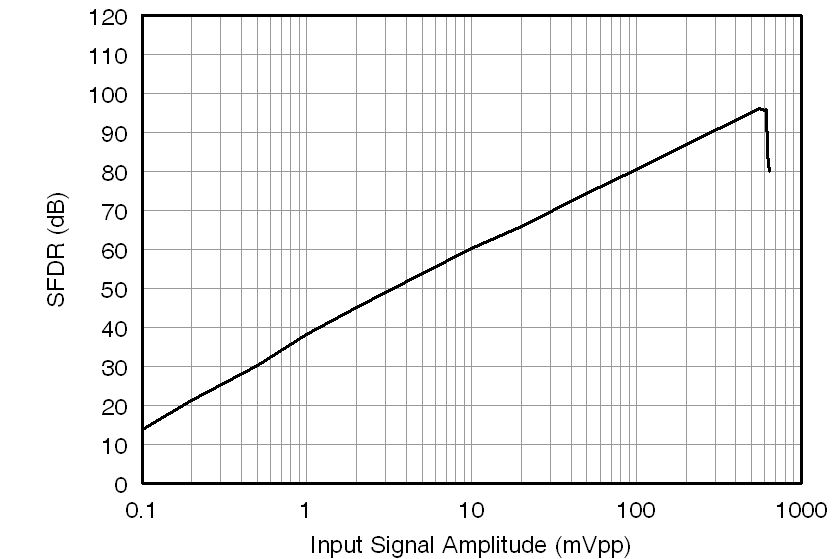Figure 31. Spurious-Free Dynamic Range vs Input Signal Amplitude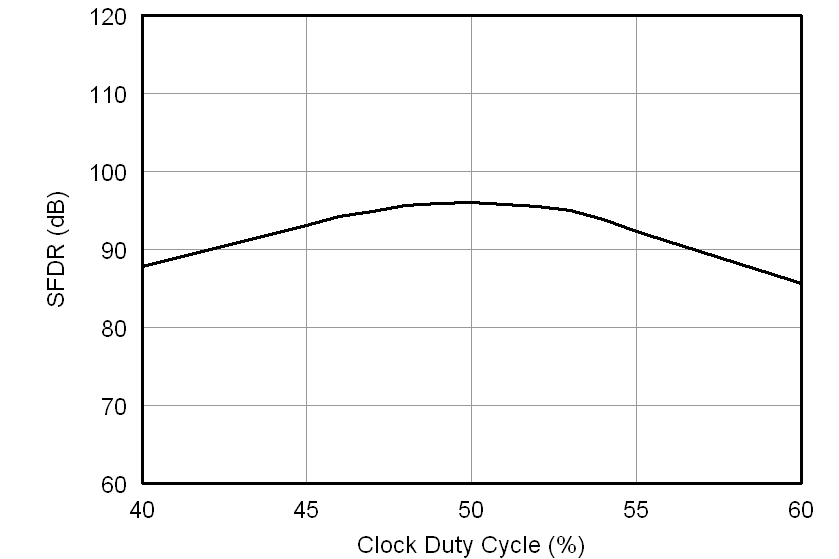Figure 33. Spurious-Free Dynamic Range vs Clock Duty Cycle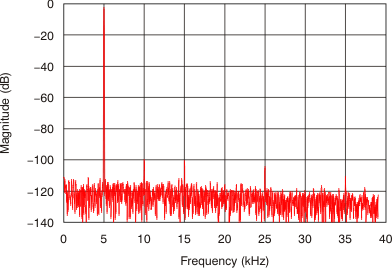Figure 35. Frequency Spectrum (4096 Point FFT, fIN = 5 kHz, 0.56 VPP)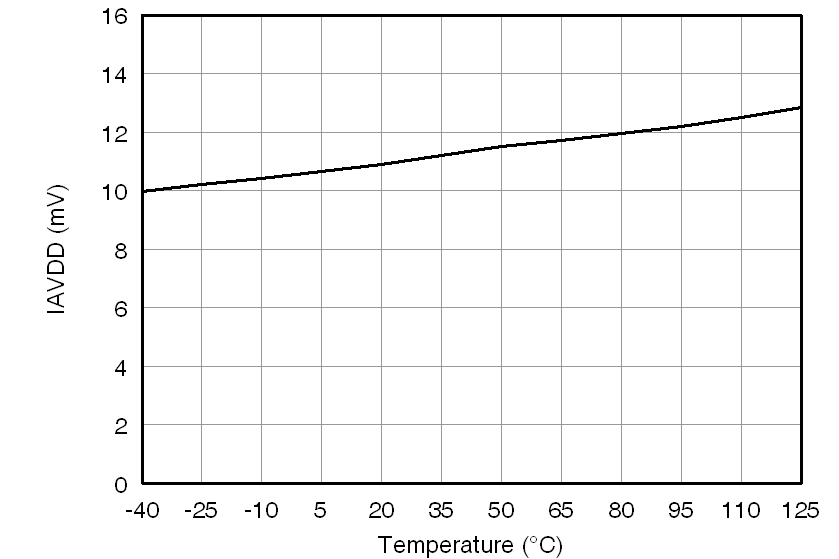Figure 37. Analog Supply Current vs Temperature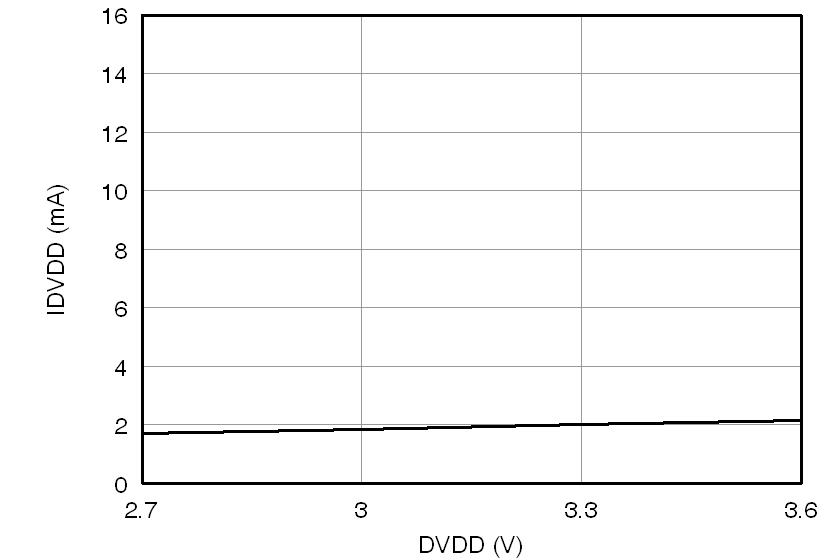Figure 39. Digital Supply Current vs Digital Supply Voltage (3 V)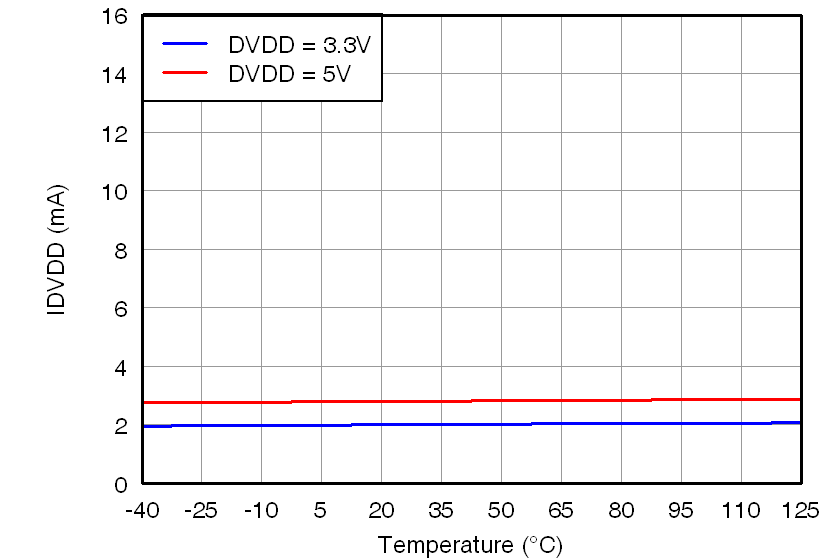Figure 41. Digital Supply Current vs Temperature